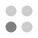• 4,284 Trophies Earned
• 217 Players Tracked
• 24 Total Trophies
• 195 Obtainable EXP
• 1,200 Points
• 162 Platinum Club
• 162 100% Club

### Pic-a-Pix Classic Trophies

•Collect all the trophies75.12%
•Solve a 5x5 puzzle99.54%
•Solve a 10x10 puzzle96.31%
•Solve a 10x10 puzzle without using an X94.01%
•Solve all the 10x10 puzzles86.64%
•Earn a medal for every 10x10 puzzle86.18%
•Solve a 15x15 puzzle89.40%
•Solve a 15x15 puzzle without using an X88.48%
•Solve all the 15x15 puzzles77.88%
•Earn a medal for every 15x15 puzzle76.96%
•Solve a 20x15 puzzle83.41%
•Solve a 20x15 puzzle without using an X82.03%
•Solve all the 20x15 puzzles76.96%
•Earn a medal for every 20x15 puzzle76.50%
•Solve a 20x20 puzzle82.95%
•Solve a 20x20 puzzle without using an X81.57%
•Solve all the 20x20 puzzles76.50%
•Earn a medal for every 20x20 puzzle76.04%
•Solve a 30x20 puzzle82.03%
•Solve a 30x20 puzzle without using an X81.57%
•Solve all the 30x20 puzzles76.96%
•Earn a medal for every 30x20 puzzle76.50%
•Solve every puzzle75.58%
•Earn a medal for every puzzle75.12%